Courses

# Notes | EduRev

## NEET : Notes | EduRev

The document Notes | EduRev is a part of the NEET Course Physics 28 Years Past year papers for NEET/AIPMT Class 12.
All you need of NEET at this link: NEET

Q.1. For a radioactive material, half-life is 10 minutes. If initially there are 600 number of nuclei, the time taken (in minutes) for the disintegration of 450 nuclei is:    (2018)
A: 20
B: 10
C: 30
D: 15
Ans:
A
Solution: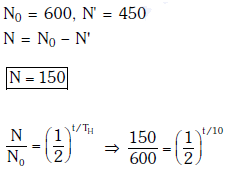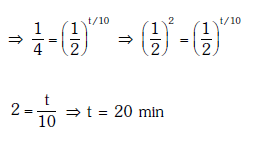Q.2. Radioactive material 'A' has decay constant '8 λ' and material 'B' has decay constant 'λ'. Initially they have same number of nuclei. After what time, the ratio of number of nuclei of material 'B' to that 'A' will be 1/e?     (2017)
A: 1/7λ
B: 1/8λ
C: 1/9λ
D: 1/10λ
Ans: A
Solution: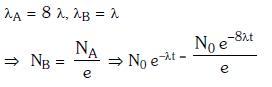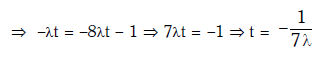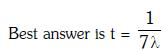Q.3. The Binding energy per nucleon of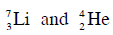nuclei are 5.60 MeV and 7.06 MeV, respectively. In the nuclear reaction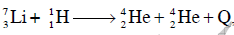the value of energy Q released is :    (2014)
A: 8.4 MeV
B: 17.3 MeV
C: 19.6 MeV
D: −2.4 MeV
Ans: B
Solution: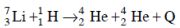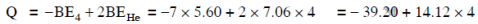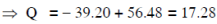Q.4. A radio isotope ‘X’ with a half life 1.4 × 109 years decays to ‘Y’ which is stable. A sample of the rock from a cave was found to contain ‘X’ and ‘Y’ in the ratio 1 : 7. The age of the rock is    (2014)
A: 4.20 × 109 years
B: 8.40 × 109 years
C: 1.96 × 109 years
D: 3.92 × 109 years
Ans: A
Solution:

Ratio of X:Y is given = 1:7
That is,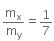⇒ 7mx = my
Let, the initial total mass is m.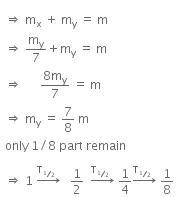Therefore, time taken to become 1/8 unstable part
= 3 x T1/2
= 3 x 1.4 x 109
= 4.2 x 109 y

Offer running on EduRev: Apply code STAYHOME200 to get INR 200 off on our premium plan EduRev Infinity!

14 docs|25 tests

,

,

,

,

,

,

,

,

,

,

,

,

,

,

,

,

,

,

,

,

,

;